# An Introduction to Lagrange Multipliers

### by Steuard Jensen

Lagrange multipliers are used in multivariable calculus to find maxima and minima of a function subject to constraints (like "find the highest elevation along the given path" or "minimize the cost of materials for a box enclosing a given volume"). It's a useful technique, but all too often it is poorly taught and poorly understood. With luck, this overview will help to make the concept and its applications a bit clearer.

Be warned: this page may not be what you're looking for! If you're looking for detailed proofs, I recommend consulting your favorite textbook on multivariable calculus: my focus here is on concepts, not mechanics. (Comes of being a physicist rather than a mathematician, I guess.) If you want to know about Lagrange multipliers in the calculus of variations, as often used in Lagrangian mechanics in physics, this page only discusses them briefly.

Here's a basic outline of this discussion:

## When are Lagrange multipliers useful?

One of the most common problems in calculus is that of finding maxima or minima (in general, "extrema") of a function, but it is often difficult to find a closed form for the function being extremized. Such difficulties often arise when one wishes to maximize or minimize a function subject to fixed outside conditions or constraints. The method of Lagrange multipliers is a powerful tool for solving this class of problems without the need to explicitly solve the conditions and use them to eliminate extra variables.

Put more simply, it's usually not enough to ask, "How do I minimize the aluminum needed to make this can?" (The answer to that is clearly "Make a really, really small can!") You need to ask, "How do I minimize the aluminum while making sure the can will hold 10 ounces of soup?" Or similarly, "How do I maximize my factory's profit given that I only have \$15,000 to invest?" Or to take a more sophisticated example, "How quickly will the roller coaster reach the ground assuming it stays on the track?" In general, Lagrange multipliers are useful when some of the variables in the simplest description of a problem are made redundant by the constraints.

## A classic example: the "milkmaid problem"

To give a specific, intuitive illustration of this kind of problem, we will consider a classic example which I believe is known as the "Milkmaid problem". It can be phrased as follows: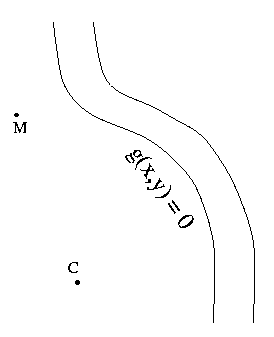It's milking time at the farm, and the milkmaid has been sent to the field to get the day's milk. She's in a hurry to get back for a date with a handsome young goatherd, so she wants to finish her job as quickly as possible. However, before she can gather the milk, she has to rinse out her bucket in the nearby river.

Just when she reaches point M, our heroine spots the cow, way down at point C. Because she is in a hurry, she wants to take the shortest possible path from where she is to the river and then to the cow. So what is the best point P on the riverbank for her to rinse the bucket? (To keep things simple, we assume that the field is flat and uniform and that all points on the river bank are equally good.)

To put this into more mathematical terms, the milkmaid wants to find the point P for which the total distance f(P) is a minimum, where f(P) is the sum of the distance d(M,P) from M to P and the distance d(P,C) from P to C (a straight line is the shortest distance between two points across a flat field). It's not quite this simple, however: if that were the whole problem, then we could just choose P anywhere on the line between M and C and the milkmaid would walk straight to the cow. But we have to impose the constraint that P is a point on the riverbank.

To express that constraint in equations, we can describe the shape of the near bank of the river as a curve satisfying some function g(x,y) = 0. (For example, if the river were a parabola we might choose g(x,y) = y - x2. If it were instead a circular lake of radius r, we might choose g(x,y) = x2 + y2 - r2.) So formally, we must minimize the function f(P) = d(M,P) + d(P, C), subject to the constraint that g(P) = 0.

## Graphical inspiration for the method

Our first way of thinking about this problem can be obtained directly from the picture itself. We want to visualize how far the milkmaid could get for any fixed total distance f(P), and use that visualization to locate the optimal point P. If we only cared about the distance of P from M, we might picture this as a set of concentric circles (curves of constant distance d(M,P): 5 meters, 10 meters, 15 meters, etc.). As soon as one of those circles was big enough to touch the river, we'd recognize the point where it touched as the closest riverbank point to M (and its radius as the minimum distance).

Of course, for most problems the curves of constant f(P) are more complicated than a bunch of concentric circles! (Often much more complicated.) But our particular example is made simpler by an obscure fact from geometry: for every point P on a given ellipse, the total distance from one focus of the ellipse to P and then to the other focus is exactly the same. (You don't need to know where this fact comes from to understand the example! But you can see it work for yourself by drawing a near-perfect ellipse with the help of two nails, a pencil, and a loop of string.)

In our problem, that means that the milkmaid could get to the cow by way of any point on a given ellipse in the same amount of time: the ellipses are curves of constant f(P). Therefore, to find the desired point P on the riverbank, we must simply find the smallest ellipse with M and C as foci that intersects the curve of the river. Just to be clear, only the "constant f(P)" property of the curves is really important; the fact that these curves are ellipses is just a lucky convenience (ellipses are easy to draw). The same idea will work no matter what shape the curves of constant f(P) happen to be.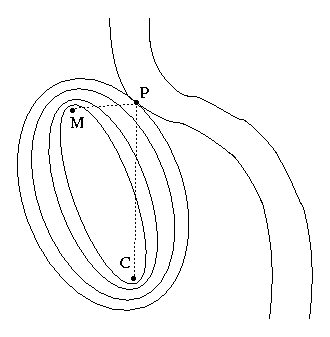The image at right shows a sequence of ellipses of larger and larger size whose foci are M and C, ending with the one that is just tangent to the riverbank. This is a very significant word! It is obvious from the picture that the "perfect" ellipse and the river are truly tangential to each other at the ideal point P. More mathematically, this means that the normal vector to the ellipse is in the same direction as the normal vector to the riverbank. A few minutes' thought about pictures like this will convince you that this fact is not specific to this problem: it is a general property whenever you have constraints. And that is the insight that leads us to the method of Lagrange multipliers.

## The mathematics of Lagrange multipliers

In multivariable calculus, the gradient of a function h (written ∇h) is a normal vector to a curve (in two dimensions) or a surface (in higher dimensions) on which h is constant: n = ∇h(P). The length of the normal vector doesn't matter: any constant multiple of ∇h(P) is also a normal vector. In our case, we have two functions whose normal vectors are parallel, so

∇f(P) = λ ∇g(P).

The unknown constant multiplier λ is necessary because the magnitudes of the two gradients may be different. (Remember, all we know is that their directions are the same.)

In D dimensions, we now have D+1 equations in D+1 unknowns. D of the unknowns are the coordinates of P (e.g. x, y, and z for D = 3), and the other is the new unknown constant λ. The equation for the gradients derived above is a vector equation, so it provides D equations of constraint. I once got stuck on an exam at this point: don't let it happen to you! The original constraint equation g(P) = 0 is the final equation in the system. Thus, in general, a unique solution exists.

As in many maximum/minimum problems, cases do exist with multiple solutions. There can even be an infinite number of solutions if the constraints are particularly degenerate: imagine if the milkmaid and the cow were both already standing right at the bank of a straight river, for example. In many cases, the actual value of the Lagrange multiplier isn't interesting, but there are some situations in which it can give useful information (as discussed below).

That's it: that's all there is to Lagrange multipliers. Just set the gradient of the function you want to extremize equal to the gradient of the constraint function. You'll get a vector's worth of (algebraic) equations, and together with the original constraint equation they determine the solution.

## A formal mathematical inspiration

There is another way to think of Lagrange multipliers that may be more helpful in some situations and that can provide a better way to remember the details of the technique (particularly with multiple constraints as described below). Once again, we start with a function f(P) that we wish to extremize, subject to the condition that g(P) = 0. Now, the usual way in which we extremize a function in multivariable calculus is to set ∇f(P) = 0. How can we put this condition together with the constraint that we have?

One answer is to add a new variable λ to the problem, and to define a new function to extremize:

F(P, λ) = f(P) - λ g(P).

(Some references call this F "the Lagrangian function". I am not familiar with that usage, although it must be related to the somewhat similar "Lagrangian" used in advanced physics.)

We next set ∇F(P, λ) = 0, but keep in mind that the gradient is now D + 1 dimensional: one of its components is a partial derivative with respect to λ. If you set this new component of the gradient equal to zero, you get the constraint equation g(P) = 0. Meanwhile, the old components of the gradient treat λ as a constant, so it just pulls through. Thus, the other D equations are precisely the D equations found in the graphical approach above.

As presented here, this is just a trick to help you reconstruct the equations you need. However, for those who go on to use Lagrange multipliers in the calculus of variations, this is generally the most useful approach. I suspect that it is in fact very fundamental; my comments about the meaning of the multiplier below are a step toward exploring it in more depth, but I have never spent the time to work out the details.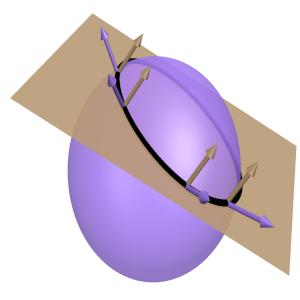## Several constraints at once

If you have more than one constraint, all you need to do is to replace the right hand side of the equation with the sum of the gradients of each constraint function, each with its own (different!) Lagrange multiplier. This is usually only relevant in at least three dimensions (since two constraints in two dimensions generally intersect at isolated points).

Again, it is easy to understand this graphically. [My thanks to Eric Ojard for suggesting this approach]. Consider the example shown at right: the solution is constrained to lie on the brown plane (as an equation, "g(P) = 0") and also to lie on the purple ellipsoid ("h(P) = 0"). For both to be true, the solution must lie on the black ellipse where the two intersect. I have drawn several normal vectors to each constraint surface along the intersection. The important observation is that both normal vectors are perpendicular to the intersection curve at each point. In fact, any vector perpendicular to it can be written as a linear combination of the two normal vectors. (Assuming the two are linearly independent! If not, the two constraints may already give a specific solution: in our example, this would happen if the plane constraint was exactly tangent to the ellipsoid constraint at a single point.)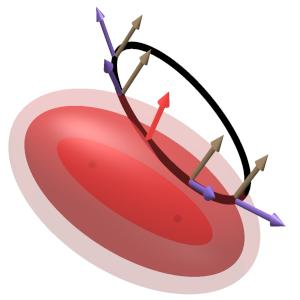The significance of this becomes clear when we consider a three dimensional analogue of the milkmaid problem. The pink ellipsoids at right all have the same two foci (which are faintly visible as black dots in the middle), and represent surfaces of constant total distance for travel from one focus to the surface and back to the other. As in two dimensions, the optimal ellipsoid is tangent to the constraint curve, and consequently its normal vector is perpendicular to the combined constraint (as shown). Thus, the normal vector can be written as a linear combination of the normal vectors of the two constraint surfaces. In equations, this statement reads

∇f(P) = λ ∇g(P) + μ ∇h(P).

just as described above. The generalization to more constraints and higher dimensions is exactly the same.

## The meaning of the multiplier

As a final note, I'll say a few words about what the Lagrange multiplier "means", in ways inspired by both physics and economics. In our mostly geometrical discussion so far, λ was just an artificial variable that lets us compare the directions of the gradients without worrying about their magnitudes. But in cases where the function f(P) and the constraint g(P) have specific meanings, the Lagrange multiplier often has an identifiable significance as well.

One example of this is inspired by the physics of forces and potential energy. In the formal approach based on the combined "Lagrangian function" F(P, λ) described two sections above, the constraint function g(P) can be thought of as "competing" with the desired function f(P) to "pull" the point P to its minimum or maximum. The Lagrange multiplier λ can be thought of as a measure of how hard g(P) has to pull in order to make those "forces" balance out on the constraint surface. (This generalizes naturally to multiple constraints, which typically "pull" in different directions.) And in fact, that word "forces" is very significant: in physics based on Lagrange multipliers in the calculus of variations (as described below) this analogy turns out to be literally true: there, λ is the force of constraint.

The Lagrange multiplier λ has meaning in economics as well. If you're maximizing profit subject to a limited resource, λ is that resource's marginal value (sometimes called the "shadow price" of the resource). Specifically, the value of the Lagrange multiplier is the rate at which the optimal value of the function f(P) changes if you change the constraint.

I don't have an immediate intuitive explanation for why this is true, but the steps of the formal proof are at least reasonably illuminating. To demonstrate this result mathematically (following the approach of this economics-inspired tutorial by Martin Osborne), write the constraint in the form "g(P) = g(x,y) = c" for some constant c. (This is mathematically equivalent to our usual g(P)=0, but allows us to easily describe a whole family of constraints. Also, I am writing this in terms of just two coordinates x and y for clarity, but the generalization to more is straightforward.) For any given value of c, we can use Lagrange multipliers to find the optimal value of f(P) and the point where it occurs. Call that optimal value f0, occurring at coordinates (x0, y0) and with Lagrange multiplier λ0. The answers we get will all depend on what value we used for c in the constraint, so we can think of these as functions of c: f0(c), x0(c), and y0(c).

To find how the optimal value changes when you change the constraint, just take the derivative: df0/dc. Of course, f(P) only depends on c because the optimal coordinates (x0, y0) depend on c: we could write it as f0(x0(c),y0(c)). So we have to use the (multivariable) chain rule:

df0/dc = ∂f0/∂x0 dx0/dc + ∂f0/∂y0 dy0/dc = ∇f0 ∙ dx0/dc

In the final step, I've suggestively written this as a dot product between the gradient of f0 and the derivative of the coordinate vector. So here's the clever trick: use the Lagrange multiplier equation to substitute ∇f = λ∇g:

df0/dc = λ0 ∇g0 ∙ dx0/dc = λ0 dg0/dc

But the constraint function is always equal to c, so dg0/dc = 1. Thus, df0/dc = λ0. That is, the Lagrange multiplier is the rate of change of the optimal value with respect to changes in the constraint.

This is a powerful result, but be careful when using it! In particular, you have to make sure that your constraint function is written in just the right way. You would get the exact same optimal value f0 whether you wrote "g(x,y) = x+y = 0" or "g(x,y) = -2x-2y = 0", but the resulting Lagrange multipliers would be quite different.

I haven't studied economic applications Lagrange multipliers myself, so if that is your interest you may want to look for other discussions from that perspective once you understand the basic idea. (The tutorial that inspired my discussion here seems reasonable.) The best way to understand is to try working examples yourself; you might appreciate this problem set introducing Lagrange multipliers in economics both for practice and to develop intuition.

## Examples of Lagrange multipliers in action

### A box of minimal surface area

What shape should a rectangular box with a specific volume (in three dimensions) be in order to minimize its surface area? (Questions like this are very important for businesses that want to save money on packing materials.) Some people may be able to guess the answer intuitively, but we can prove it using Lagrange multipliers.

Let the lengths of the box's edges be x, y, and z. Then the constraint of constant volume is simply g(x,y,z) = xyz - V = 0, and the function to minimize is f(x,y,z) = 2(xy+xz+yz). The method is straightforward to apply:

2<y+z, x+z, x+y> = ∇f(x,y,z) = λ ∇g(x,y,z) = λ <yz, xz, xy>.

(The angle bracket notation <a,b,c> is one conventional way to denote a vector.) Now just solve those three equations; the solution is x = y = z = 4/λ. We could eliminate λ from the problem by using xyz = V, but we don't need to: it is already clear that the optimal shape is a cube.

### The closest approach of a line to a point

This example isn't the perfect illustration of where Lagrange multiples are useful, since it is fairly easy to solve without them and not all that convenient to solve with them. But it's a very simple idea, and because of a careless mistake on my part it was the first example that I applied the technique to. Here's the story...

When I first took multivariable calculus (and before we learned about Lagrange multipliers), my teacher showed the example of finding the point P = <x,y> on a line (y = m x + b) that was closest to a given point Q = <x0,y0>. The function to minimize is of course

d(P,Q) = sqrt[(x-x0)² + (y-y0)²].

(Here, "sqrt" means "square root", of course; that's hard to draw in plain text.)

The teacher went through the problem on the board in the most direct way (I'll explain it later), but it was taking him a while and I was a little bored, so I idly started working the problem myself while he talked. I just leapt right in and set ∇d(x,y) = 0, so

<x-x0, y-y0> / sqrt[(x-x0)² + (y-y0)²] = <0,0>,

and thus x = x0 and y = y0. My mistake here is obvious, so I won't blame you for having a laugh at my expense: I forgot to impose the constraint that <x,y> be on the line! (In my defense, I wasn't really focusing on what I was doing, since I was listening to lecture at the same time.) I felt a little silly, but I didn't think much more about it.

Happily, we learned about Lagrange multipliers the very next week, and I immediately saw that my mistake had been a perfect introduction to the technique. We write the equation of the line as g(x,y) = y - m x - b = 0, so ∇g(x,y) = <-m,1>. So we just set the two gradients equal (up to the usual factor of λ), giving

<x-x0, y-y0> / sqrt[(x-x0)² + (y-y0)²] = λ<-m,1>.

The second component of this equation is just an equation for λ, so we can substitute that value for λ into the first component equation. The denominators are the same and cancel, leaving just (x-x0) = -m(y-y0). Finally, we substitute y = m x+b, giving x-x0 = -m² x - m b + m y0, so we come to the final answer: x = (x0 + m y0 - m b) / (m² + 1). (And thus y = (m x0 + m² y0 + b)/(m² + 1).)

So what did my teacher actually do? He used the equation of the line to substitute y for x in d(P,Q), which left us with an "easy" single-variable function to deal with... but a rather complicated one:

d(P,Q) = sqrt[(x - x0)² + (m x + b - y0)²]

To solve the problem from this point, you take the derivative and set it equal to zero as usual. It's a bit of a pain, since the function is a mess, but the answer is x = (x0 + m y0 - m b)/(m² + 1). That's exactly what we got earlier, so both methods seem to work. In this case, the second method may be a little faster (though I didn't show all of the work), but in more complicated problems Lagrange multipliers are often much easier than the direct approach.

Finally, I've recorded a couple of video examples of solving problems using Lagrange multipliers. They're far from perfect, and they're aimed more at problem solving than explaining the concepts, but some folks may be interested. The first does try to explain the concepts a little in the context of finding the closest point to the origin on a parabola. Another is purely problem solving, ostensibly about finding the nearest water to a house:

I've also recorded a video example involving a (somewhat artificial) commodities trading firm with five parameters and two constraints:

## Lagrange multipliers in the calculus of variations (often in physics)

This section will be brief, in part because most readers have probably never heard of the calculus of variations. Many people first see this idea in advanced physics classes that cover Lagrangian mechanics, and that will be the perspective taken here (in particular, I will use variable names inspired by physics). If you don't already know the basics of this subject (specifically, the Euler-Lagrange equations), you'll probably want to just skip this section.

The calculus of variations is essentially an extension of calculus to the case where the basic variables are not simple numbers xi (which can be thought of as a position) but functions xi(t) (which in physics corresponds to a position that changes in time). Rather than seeking the numbers xi that extremize a function f(xi), we seek the functions xi(t) that extremize the integral (dt) of a function L[xi(t), xi'(t), t], where xi'(t) are the time derivatives of xi(t). (The reason we have to integrate first is to get an ordinary number out: we know what "maximum" and "minimum" mean for numbers, but there could be any number of definitions of those concepts for functions.) In most cases, we integrate between fixed values t0 and t1, and we hold the values xi(t0) and xi(t1) fixed. (In physics, that means that the initial and final positions are held constant, and we're interested finding the "best" path to get between them; L defines what we mean by "best".)

The solutions to this problem can be shown to satisfy the Euler-Lagrange equations (I have suppressed the "(t)" in the functions xi(t):

∂L/∂xi - d/dt ( ∂L/∂xi' ) = 0.

(Note that the derivative d/dt is a total derivative, while the derivatives with respect to xi and xi' are "partials", at least formally.)

Imposing constraints on this process is often essential. In physics, it is common for an object to be constrained on some track or surface, or for various coordinates to be related (like position and angle when a wheel rolls without slipping, as discussed below). To do this, we follow a simple generalization of the procedure we used in ordinary calculus. First, we write the constraint as a function set equal to zero: g(xi, t) = 0. (Constraints that necessarily involve the derivatives of xi often cannot be solved.) And second, we add a term to the function L that is multiplied by a new function λ(t): Lλ[xixi', λ, t] = L[xixi', t] + λ(t) g(xi, t).

From here, we proceed exactly as you would expect: λ(t) is treated as another coordinate function, just as λ was treated as an additional coordinate in ordinary calculus. The Euler-Lagrange equations are then written as

∂L/∂xi - d/dt (∂L/∂xi') + λ(t) (∂g/∂xi) = 0.

This can be generalized to the case of multiple constraints precisely as before, by introducing additional Lagrange multiplier functions like λ. There are further generalizations possible to cases where the constraint(s) are linear combinations of derivatives of the coordinates (rather than the coordinates themselves), but I won't go into that much detail here.

As mentioned in the calculus section, the meaning of the Lagrange multiplier function in this case is surprisingly well-defined and can be quite useful. It turns out that Qi = λ(t) (∂g/∂x i) is precisely the force required to impose the constraint g(xi, t) (in the "direction" of xi). This is fairly natural: the constraint term (λ g) added to the Lagrangian plays the same role as a (negative) potential energy -Vconstraint, so we can compute the resulting force as ∇(-Vconstr) = λ ∇g in something reminiscent of the usual way. Thus, for example, Lagrange multipliers can be used to calculate the force you would feel while riding a roller coaster. If you want this information, Lagrange multipliers are one of the best ways to get it.

## An example: rolling without slipping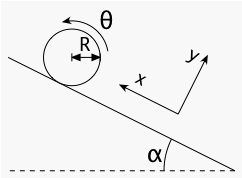One of the simplest applications of Lagrange multipliers in the calculus of variations is a ball (or other round object) rolling down a slope without slipping in one dimension. (As usual, a problem this simple can probably be solved just as easier by other means, but it still illustrates the idea.) Let the ball have mass M, moment of inertia I, and radius R, and let the angle of the slope be α. We choose the coordinate x to point up the slope and the coordinate θ to show rotation in the direction that would naturally go in that same direction, so that the "rolling without slipping" condition is x = R θ. Both x and θ are functions of time, x(t) and θ(t), but for clarity I will not write that dependence explicitly.

In general, the kinetic energy T of an object undergoing both translational and rotational motion is T = ½ M (x')2 + ½ I (θ')2. (As before, the prime (') denotes a time derivative, so this is a function of velocity and angular velocity.) Meanwhile, the potential energy V of the ball can be written as V = M g h = M g x sin α. Thus, the Lagrangian for the system is

L = T - V = ½ M (x')2 + ½ I (θ')2 - M g x sin α.

If we solved the Euler-Lagrange equations for this Lagrangian as it stands, we would find that x(t) described the ball sliding down the slope with constant acceleration in the absence of friction while θ(t) described the ball rotating with constant angular velocity: the rotational motion and translational motion are completely independent (and no torques act on the system). To impose the condition of rolling without slipping, we use a Lagrange multiplier function λ(t) to force the constraint function G(x,θ,t) = x - R θ to vanish:

L = ½ M (x')2 + ½ I (θ')2 - M g x sin α + λ (x - R θ).

(That is, λ is multiplying the constraint function, as usual.) We now find the Euler-Lagrange equations for each of the three functions of time: x, θ, and λ. Respectively, the results are:

M x'' + M g sin α - λ = 0
I θ'' + R λ = 0,       and
x - R θ = 0.

It is then straightforward to solve for the three "unknowns" in these equations:

x'' = -g sin α  M R2 / (M R2 + I)
θ'' = -g sin α  M R / (M R2 + I)
λ = M g sin α  I / (M R2 + I).

The first two equations give the constant acceleration and angular acceleration experienced as the ball rolls down the slope. And the equation for λ is used in finding the forces that implement the constraint in the problem. Specifically, these are the force and torque due to friction felt by the ball:

Fx = λ ∂G/∂x = M g sin α  I / (M R2 + I)
τ = λ ∂G/∂θ = -R M g sin α  I / (M R2 + I).

Looking at these results, we can see that the force of friction is positive: it points in the +x direction (up the hill) which means that it is slowing down the translational motion. But the torque is negative: it acts in the direction corresponding to rolling down the hill, which means that the speed of rotation increases as the ball rolls down. That is exactly what we would expect!

As a final note, you may be worried that we would get different answers for the forces of constraint if we just normalized the constraint function G(x,θ,t) differently (for example, if we set G = 2 x - 2 R θ). Happily, that will not end up affecting the final answers at all. A change in normalization for G will lead to a different answer for λ (e.g. exactly half of what we found above), but the products of λ and the derivatives of G will remain the same.

## Final thoughts

This page certainly isn't a complete explanation of Lagrange multipliers, but I hope that it has at least clarified the basic idea a little bit. I'm always glad to hear constructive criticism and positive feedback, so feel free to write to me with your comments. (My thanks to the many people whose comments have already helped me to improve this presentation.) I hope that I have helped to make this extremely useful technique make more sense. Best wishes using Lagrange multipliers in the future!

Up to my tutorial page.
Up to my teaching page.
Up to my professional page.
My personal site is also available.

Any questions or comments? Write to me: jensens@alma.edu